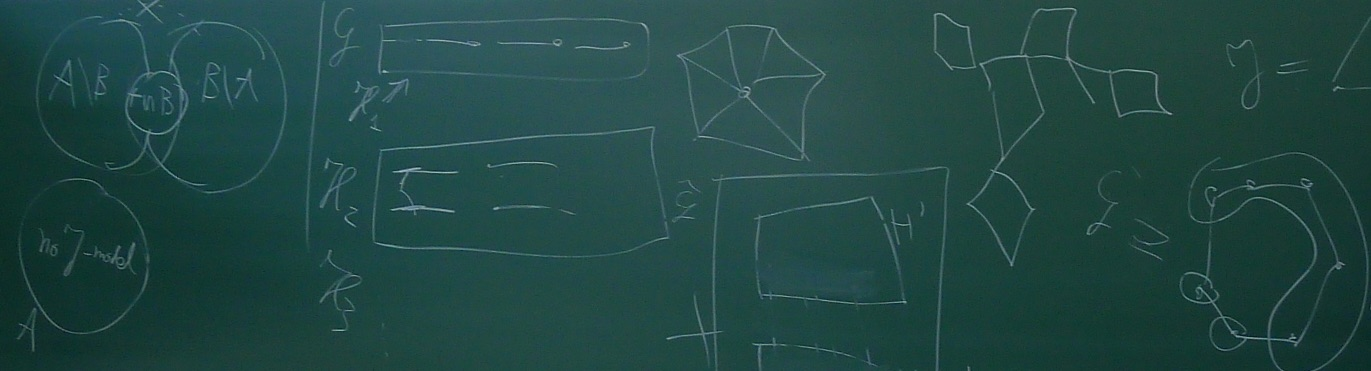A (Mini-)Symposium on GraphsTuesday, 26 June 2018

We were delighted to host the following set of talks on the campus of Radboud University Nijmegen.

### Programme

 13:15 - 13:45 Wouter Cames van Batenburg A tight Erdős-Pósa function for planar minors  ± Let $$H$$ be a planar graph. We prove that there exists a constant $$c:=c(H)$$, such that for all $$k \in \mathbb N$$ and all graphs $$G$$, either $$G$$ contains $$k$$ vertex-disjoint subgraphs each containing $$H$$ as a minor, or there is a subset $$X$$ of at most $$c k \log k$$ vertices such that $$G-X$$ has no $$H$$ minor. This bound is best possible, up to the value of $$c$$, and improves upon the bounds of Robertson and Seymour, and of Chekuri and Chuzhoy. Joint work with Tony Huynh, Gwenaël Joret and Jean-Florent Raymond. 13:45 - 14:35 Gwenaël Joret Seymour's conjecture on 2-connected graphs of large pathwidth  ± In the first paper of their graph minors series, Robertson and Seymour proved that for every forest $$F$$, there exists a constant $$p$$ such that every graph with pathwidth at least $$p$$ contains $$F$$ as a minor. Since forests have unbounded pathwidth, this implies that a minor-closed class of graphs has unbounded pathwidth if and only if it includes all forests. However, these certificates of large pathwidth are not 2-connected, so it is natural to ask for which minor-closed classes $$C$$, does every 2-connected graph in $$C$$ have bounded pathwidth? In 1993, Paul Seymour proposed the following answer. A graph $$H$$ is an apex-forest if $$H-v$$ is a forest for some vertex $$v$$ of $$H$$. A graph is outerplanar if it has an embedding in the plane with all the vertices on the outerface. These two graph classes are relevant since they both contain 2-connected graphs with arbitrarily large pathwidth. Seymour conjectured the following converse holds: For every apex-forest $$H_1$$ and outerplanar graph $$H_2$$ there is an integer $$p$$ such that every 2-connected graph of pathwidth at least $$p$$ contains $$H_1$$ or $$H_2$$ as a minor. In this talk I will outline a proof of this conjecture obtained jointly with Tony Huynh, Piotr Micek, and David R. Wood. An independent proof was recently obtained by Dang and Thomas. 14:35 - 14:50 Coffee/tea 14:50 - 15:40 Tobias Müller Logic and random graphs  ± We say that a graph property is expressible in the "first order language of graphs" if it can be written as a logic sentence using the universal and existential quantifiers with variables ranging over the nodes of the graph, the usual connectives AND, OR, NOT, parentheses and the relations = and ~, where x ~ y means that x and y share an edge. For example, the property that G contains a triangle can be written as Exists x,y,z : (x ~ y) AND (x ~ z) AND (y ~ z). A classical result of Glebskii et al. 1969 and indepently Fagin 1976 states that if one samples a graph on n vertices uniformly at random from all such graphs then every first order expressible graph property holds with probability tending to either zero or one (as n tends to infinity). Since then, first order expressible properties have been studied extensively on the most commonly studied model of random graphs, the Erdos-Renyi model. A number of very attractive and surprising results have been obtained, and by now we have a fairly full description of the behaviour of first order expressible properties on this model. After describing some of these results, time permitting, I will discuss some recent and ongoing work where we try to see what happens both in other models of random graphs (such as random planar graphs, random perfect graphs, random geometric graphs, ...) and when one considers other (i.e. "higher order") logics. (Based on joint works with S. Haber, P. Heinig, M. Noy, A. Taraz) 15:40 - 16:30 Alex Scott Induced trees and Erdős-Hajnal  ± The Erdős-Hajnal Conjecture states that for every graph $$F$$ there is a constant $$c>0$$ such that every graph on $$n$$ vertices with no induced copy of $$F$$ contains a clique or stable set of size at least $$n^c$$. The conjecture is known to hold for only a few graphs $$F$$, but there has been recent progress in showing that it holds when both a graph $$F$$ and its complement are excluded. We prove a conjecture of Liebenau, Pilipczuk, Seymour and Spirkl, that this holds whenever $$F$$ is a tree. Joint work with Maria Chudnovsky, Paul Seymour and Sophie Spirkl. 16:30 - 16:40 Break 16:40 - 17:30 Jean-Sébastien Sereni Colour space and number of 3-colourings  ± I will present an algebraic approach introduced by Jensen and Thomassen to study the number of colourings of a graph, more specifically to obtain sufficient conditions for a graph to have an exponential number of them (in terms of the number of vertices). Several conjectures will be considered, and we'll focus particularly on a conjecture of Thomassen that every triangle-free planar graph has an exponential number of 3-colourings. The talk is based on a joint work with S. Norin and a joint work with Z. Dvořák.

The event was organised in connection with the PhD defence of Wouter Cames van Batenburg the following day.

Congratulations Dr. Cames van Batenburg!

Eric Cator and Ross Kang were Wouter's supervisors, and by coincidence were also the organisers of this mini-symposium.

Last modification: